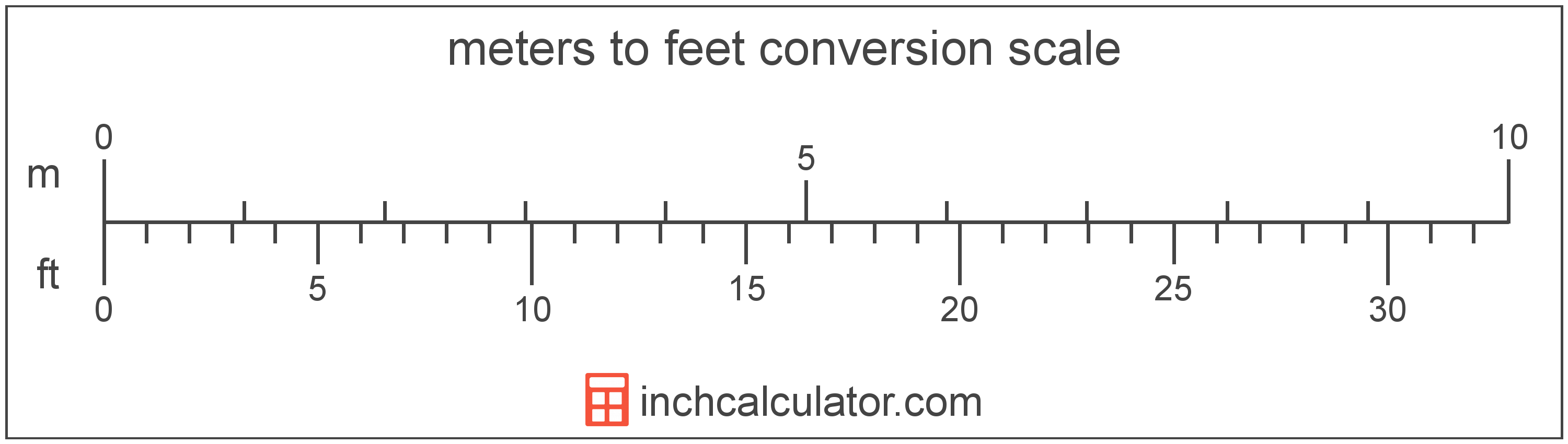# Feet to Meters Converter

Enter the length in feet below to get the value converted to meters.

ft
in
Results in Meters:1 ft = 0.3048 m

Do you want to convert meters to feet?

## How to Convert Feet to Meters

To convert a measurement in feet to a measurement in meters, multiply the length by the following conversion ratio: 0.3048 meters/foot.

Since one foot is equal to 0.3048 meters, you can use this simple formula to convert:

meters = feet × 0.3048

The length in meters is equal to the length in feet multiplied by 0.3048.

For example, here's how to convert 5 feet to meters using the formula above.
meters = (5' × 0.3048) = 1.524 m### How Many Meters Are in a Foot?

There are 0.3048 meters in a foot, which is why we use this value in the formula above.

1' = 0.3048 m

### Quick Tip:

To quickly convert feet to meters in your head, without a calculator, you can divide the measurement in feet by 3 to get a rough approximation. For instance, to approximately convert 12 feet to meters in your head, divide it by 3, which is roughly 4 meters (12 ÷ 3 ≈ 4).

Note that this is not a precise method, but rather a quick shortcut for a rough conversion on the fly when you don't have a calculator handy.

## How to Convert Feet and Inches to Meters

Instead of decimal form, feet are often expressed with the decimal portion in inches. To convert feet and inches to meters, you'll need to convert to feet in decimal form first.

To do this, convert the inches portion to feet by dividing it by 12. The result should be a decimal smaller than 1.

Then, add that to the feet portion to get the result in feet in decimal form.

Finally, convert feet to meters using the method shown above.

For example, let's find the height of a person that is 5' 9" tall in meters.

Start by converting the inches portion to decimal feet by dividing 9" by 12. 9" divided by 12 is 0.75 feet.

9 in ÷ 12 = 0.75 ft

Then, add that to the number of whole feet, which is 5.75 ft.

5 + 0.75 = 5.75

Finally, use the feet to meters formula above to complete the conversion.

5.75 × 0.3048 = 1.7526 m

Thus, the height of a person that is 5' 9" tall in meters is 1.7526 m. You can also use our height converter for this.

## What is a Foot?

The foot is a unit of length measurement equal to 12 inches or 1/3 of a yard. Because the international yard is legally defined to be equal to exactly 0.9144 meters, one foot is equal to 0.3048 meters.

The foot is a US customary and imperial unit of length. Feet can be abbreviated as ft; for example, 1 foot can be written as 1 ft.

Feet can also be denoted using the symbol, otherwise known as a prime, though a single-quote (') is often used instead of the prime symbol for convenience. Using the prime symbol, 1 ft can be written as 1′.

Measurements in feet are most commonly taken using either a standard 12" ruler or a tape measure, though there are many other measuring devices available. Feet are sometimes referred to as linear feet, which are simply a measurement of length in feet.

You might be interested in our feet and inches calculator, which can add feet with other units of measurement such as inches, centimeters, or meters.

## What is a Meter?

According to the most recent 2019 definition, the meter is defined as the distance traveled by light in vacuum during a time interval with a duration of 1/299,792,458 of a second. One meter is equal to 100 centimeters, 3.28084 feet, or 39.37 inches.

The meter, or metre, is the SI base unit for length in the metric system. Meters can be abbreviated as m; for example, 1 meter can be written as 1 m.

We recommend using a ruler or tape measure for measuring length, which can be found at a local retailer or home center. Rulers are available in imperial, metric, or a combination of both values, so make sure you get the correct type for your needs.

Need a ruler? Try our free downloadable and printable rulers, which include both imperial and metric measurements.

## Foot to Meter Conversion Table

Table showing various foot measurements converted to meters.
Feet Meters
1' 0.3048 m
2' 0.6096 m
3' 0.9144 m
4' 1.2192 m
5' 1.524 m
6' 1.8288 m
7' 2.1336 m
8' 2.4384 m
9' 2.7432 m
10' 3.048 m
11' 3.3528 m
12' 3.6576 m
13' 3.9624 m
14' 4.2672 m
15' 4.572 m
16' 4.8768 m
17' 5.1816 m
18' 5.4864 m
19' 5.7912 m
20' 6.096 m
21' 6.4008 m
22' 6.7056 m
23' 7.0104 m
24' 7.3152 m
25' 7.62 m
26' 7.9248 m
27' 8.2296 m
28' 8.5344 m
29' 8.8392 m
30' 9.144 m
31' 9.4488 m
32' 9.7536 m
33' 10.06 m
34' 10.36 m
35' 10.67 m
36' 10.97 m
37' 11.28 m
38' 11.58 m
39' 11.89 m
40' 12.19 m

## References

1. National Institute of Standards and Technology, U.S. Survey Foot: Revised Unit Conversion Factors, https://www.nist.gov/pml/us-surveyfoot/revised-unit-conversion-factors
2. International Bureau of Weights and Measures, The International System of Units, 9th Edition, 2019, https://www.bipm.org/documents/20126/41483022/SI-Brochure-9-EN.pdf